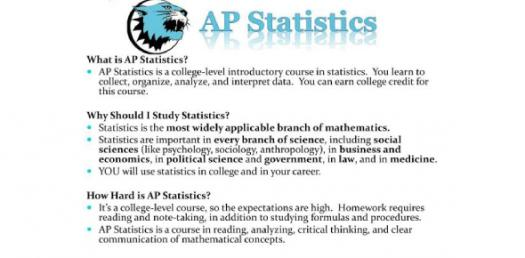# Test Yourself! AP Statistics Trivia Questions Quiz

5 Questions | Total Attempts: 603Settings.

Related Topics
• 1.
The following boxplots summarize two data sets, X and Y. Which of the following must be true?
• A.

Set X and set Y have the same number of data points.

• B.

The shaded box of set X contains more data points than the shaded box of set Y.

• C.

The data set X have a larger range than the data in set Y.

• D.

About 50% of the values in set X are greater than about 75% of the values in set Y.

• E.

The median of set X is less than the median of set Y.

• 2.
Two measures, x and y, are taken on numerous subjects, and a least squares regression equation is computed. The resulting equation is predicted y = 382.1 - 12.25x. A correct interpretation for the slope and intercept is:
• A.

For every increase of 100 units in x, y increases approximately 1225 units; when x = 0, y is predicted to be 382.1.

• B.

For every increase of 100 units in x, y decreases approximately 1225 units; when x = 0, y is predicted to be 382.1.

• C.

For every increase of 100 units in x, y increases approximately 32,810 units; when x = 0, y is predicted to be 12.25.

• D.

For every increase of 100 units in x, y decreases approximately 1225 units; when x = 0, y is predicted to be 12.25.

• E.

For every increase of 100 units in x, y increases approximately 1225 units; when x = 0, y is predicted to be -12.25.

• 3.
Which of the following sampling designs does not contain a source of bias?
• A.

A politician would like to know how her constituents feel about a particular issue. As a result, her office mails questionnaires about the issue to a random sample of adults in her political district.

• B.

A company uses the telephone directory to randomly select adults for a telephone survey to gauge their feelings toward items manufactured by the company.

• C.

An interviewer selects a random sample of individuals to question about a particular issue. Since some of the individuals are not informed about the issue, the interviewer gives background and his personal view on the issue before recording their responses.

• D.

A news show asks viewers to call a toll-free number to express their opinions about a recent high-publicity trial.

• E.

One thousand numbered tickets are sold as a fund-raiser. Five numbers are chosen randomly, and the individuals with the winning ticket numbers each win \$10.

• 4.
A class of statistics students scored the following grades on a ten point quiz:  5, 2, 4, 6, 7, 9, 8, 10, 10, 6, 7, 7, 10, 4, 2.  Their teacher, who sets a passing grade at 70%, looks at the distribution and see that it is
• A.

Skewed right with about 50% of the class passing.

• B.

Skewed left with about 50% of the class passing.

• C.

Skewed right with about 50% of the class failing.

• D.

Nearly symmetric with one outlier.

• E.

Nearly symmetric with no outliers.

• 5.
Suppose that 25% of horses live over 23.4 years, while 85% live less than 25.2 years.  Assuming the ages of horses are normally distributed, what are the mean and standard deviation for the life expectancy of horses?
• A.

20.14 and 4.86

• B.

22.69 and 1.052

• C.

22.69 and 4.97

• D.

24.11 and 1.052

• E.

Not enough information is given to find the mean and s.d.